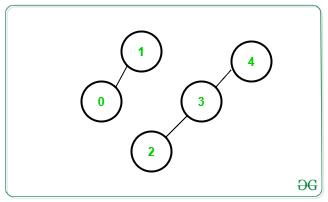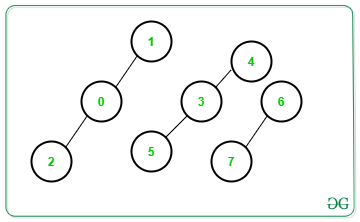# Number of connected components of a graph ( using Disjoint Set Union )

• Difficulty Level : Hard
• Last Updated : 28 Jun, 2022

Given an undirected graph G with vertices numbered in the range [0, N] and an array Edges[][] consisting of M edges, the task is to find the total number of connected components in the graph using Disjoint Set Union algorithm.

Examples:

Input: N = 4, Edges[][] = {{1, 0}, {2, 3}, {3, 4}}
Output: 2
Explanation: There are only 2 connected components as shown below:Input: N = 4, Edges[][] = {{1, 0}, {0, 2}, {3, 5}, {3, 4}, {6, 7}}
Output: 3
Explanation: There are only 3 connected components as shown below:Approach: The problem can be solved using Disjoint Set Union algorithm. Follow the steps below to solve the problem:

• In DSU algorithm, there are two main functions, i.e. connect() and root() function.
• connect(): Connects an edge.
• root(): Recursively determine the topmost parent of a given edge.
• For each edge {a, b}, check if a is connected to b or not. If found to be false, connect them by appending their top parents.
• After completing the above step for every edge, print the total number of the distinct top-most parents for each vertex.

Below is the implementation of the above approach:

## C++

 `// C++ program for the above approach` `#include ``using` `namespace` `std;` `// Stores the parent of each vertex``int` `parent;` `// Function to find the topmost``// parent of vertex a``int` `root(``int` `a)``{``    ``// If current vertex is``    ``// the topmost vertex``    ``if` `(a == parent[a]) {``        ``return` `a;``    ``}` `    ``// Otherwise, set topmost vertex of``    ``// its parent as its topmost vertex``    ``return` `parent[a] = root(parent[a]);``}` `// Function to connect the component``// having vertex a with the component``// having vertex b``void` `connect(``int` `a, ``int` `b)``{``    ``// Connect edges``    ``a = root(a);``    ``b = root(b);` `    ``if` `(a != b) {``        ``parent[b] = a;``    ``}``}` `// Function to find unique top most parents``void` `connectedComponents(``int` `n)``{``    ``set<``int``> s;` `    ``// Traverse all vertices``    ``for` `(``int` `i = 0; i < n; i++) {` `        ``// Insert all topmost``        ``// vertices obtained``        ``s.insert(root(parent[i]));``    ``}` `    ``// Print count of connected components``    ``cout << s.size() << ``'\n'``;``}` `// Function to print answer``void` `printAnswer(``int` `N,``                 ``vector > edges)``{` `    ``// Setting parent to itself``    ``for` `(``int` `i = 0; i <= N; i++) {``        ``parent[i] = i;``    ``}` `    ``// Traverse all edges``    ``for` `(``int` `i = 0; i < edges.size(); i++) {``        ``connect(edges[i], edges[i]);``    ``}` `    ``// Print answer``    ``connectedComponents(N);``}` `// Driver Code``int` `main()``{``    ``// Given N``    ``int` `N = 8;` `    ``// Given edges``    ``vector > edges = {``        ``{ 1, 0 }, { 0, 2 }, { 5, 3 }, { 3, 4 }, { 6, 7 }``    ``};` `    ``// Function call``    ``printAnswer(N, edges);` `    ``return` `0;``}`

## Java

 `// Java program for the above approach``import` `java.util.*;``class` `GFG{` `// Stores the parent of each vertex``static` `int` `[]parent = ``new` `int``[``1000000``];` `// Function to find the topmost``// parent of vertex a``static` `int` `root(``int` `a)``{``  ` `    ``// If current vertex is``    ``// the topmost vertex``    ``if` `(a == parent[a])``    ``{``        ``return` `a;``    ``}` `    ``// Otherwise, set topmost vertex of``    ``// its parent as its topmost vertex``    ``return` `parent[a] = root(parent[a]);``}` `// Function to connect the component``// having vertex a with the component``// having vertex b``static` `void` `connect(``int` `a, ``int` `b)``{``  ` `    ``// Connect edges``    ``a = root(a);``    ``b = root(b);` `    ``if` `(a != b) {``        ``parent[b] = a;``    ``}``}` `// Function to find unique top most parents``static` `void` `connectedComponents(``int` `n)``{``    ``HashSet s = ``new` `HashSet();` `    ``// Traverse all vertices``    ``for` `(``int` `i = ``0``; i < n; i++)``    ``{` `        ``// Insert all topmost``        ``// vertices obtained``        ``s.add(parent[i]);``    ``}` `    ``// Print count of connected components``    ``System.out.println(s.size());``}` `// Function to print answer``static` `void` `printAnswer(``int` `N,``int` `[][] edges)``{` `    ``// Setting parent to itself``    ``for` `(``int` `i = ``0``; i <= N; i++)``    ``{``        ``parent[i] = i;``    ``}` `    ``// Traverse all edges``    ``for` `(``int` `i = ``0``; i < edges.length; i++)``    ``{``        ``connect(edges[i][``0``], edges[i][``1``]);``    ``}` `    ``// Print answer``    ``connectedComponents(N);``}` `// Driver Code``public` `static` `void` `main(String[] args)``{``  ` `    ``// Given N``    ``int` `N = ``8``;` `    ``// Given edges``   ``int` `[][]edges = {{ ``1``, ``0` `}, { ``0``, ``2` `},``                    ``{ ``5``, ``3` `}, { ``3``, ``4` `},``                    ``{ ``6``, ``7` `}};` `    ``// Function call``    ``printAnswer(N, edges);``}``}` `// This code is contributed by 29AjayKumar`

## Python3

 `# Python3 program for the above approach``from` `collections ``import` `defaultdict``# Given N``N ``=` `8` `# Given edges``edges ``=` `[[``1``, ``0` `], [ ``0``, ``2` `], [ ``5``, ``3` `], [ ``3``, ``4` `], [ ``6``, ``7` `]]` `# Stores the parent of each vertex``parent ``=` `list``(``range``(N))`` ` `# Function to find the topmost``# parent of vertex x``def` `find(x):``  ``if` `x !``=` `parent[x]:``    ``parent[x] ``=` `find(parent[x])``  ``return` `parent[x]` `def` `union(x,y):``  ``parent_x ``=` `find(x)``  ``parent_y ``=` `find(y)``  ``if` `parent_x !``=` `parent_y:``    ``parent[parent_y] ``=` `parent_x``    ` `for` `x,y ``in` `edges:``  ``union(x,y)` `dict_pair ``=` `defaultdict(``list``)` `for` `idx, val ``in` `enumerate``(parent):``  ``dict_pair[find(val)].append(idx)` `print``(``len``(dict_pair.keys()))``    `   `# This code is contributed by Shivam Dwivedi`

## C#

 `// C# program for the above approach``using` `System;``using` `System.Collections.Generic;``class` `GFG {``    ` `    ``// Stores the parent of each vertex``    ``static` `int``[] parent = ``new` `int``;``      ` `    ``// Function to find the topmost``    ``// parent of vertex a``    ``static` `int` `root(``int` `a)``    ``{``        ``// If current vertex is``        ``// the topmost vertex``        ``if` `(a == parent[a]) {``            ``return` `a;``        ``}``      ` `        ``// Otherwise, set topmost vertex of``        ``// its parent as its topmost vertex``        ``return` `parent[a] = root(parent[a]);``    ``}``      ` `    ``// Function to connect the component``    ``// having vertex a with the component``    ``// having vertex b``    ``static` `void` `connect(``int` `a, ``int` `b)``    ``{``        ``// Connect edges``        ``a = root(a);``        ``b = root(b);``      ` `        ``if` `(a != b) {``            ``parent[b] = a;``        ``}``    ``}``      ` `    ``// Function to find unique top most parents``    ``static` `void` `connectedComponents(``int` `n)``    ``{``        ``HashSet<``int``> s = ``new` `HashSet<``int``>();``      ` `        ``// Traverse all vertices``        ``for` `(``int` `i = 0; i < n; i++) {``      ` `            ``// Insert all topmost``            ``// vertices obtained``            ``s.Add(parent[i]);``        ``}``      ` `        ``// Print count of connected components``        ``Console.WriteLine(s.Count);``    ``}``      ` `    ``// Function to print answer``    ``static` `void` `printAnswer(``int` `N, List > edges)``    ``{``      ` `        ``// Setting parent to itself``        ``for` `(``int` `i = 0; i <= N; i++) {``            ``parent[i] = i;``        ``}``      ` `        ``// Traverse all edges``        ``for` `(``int` `i = 0; i < edges.Count; i++) {``            ``connect(edges[i], edges[i]);``        ``}``      ` `        ``// Print answer``        ``connectedComponents(N);``    ``}  ` `  ``// Driver code``  ``static` `void` `Main() {``      ` `    ``// Given N``    ``int` `N = 8;``  ` `    ``// Given edges``    ``List> edges = ``new` `List>();``    ``edges.Add(``new` `List<``int``> { 1, 0 });``    ``edges.Add(``new` `List<``int``> { 0, 2 });``    ``edges.Add(``new` `List<``int``> { 5, 3 });``    ``edges.Add(``new` `List<``int``> { 3, 4 });``    ``edges.Add(``new` `List<``int``> { 6, 7 });``  ` `    ``// Function call``    ``printAnswer(N, edges);``  ``}``}` `// This code is contributed by divyeshrabadiya07`

## Javascript

 ``

Output

`3`

Time Complexity: O(NLOGN+M)
Auxiliary Space: O(N+M)

My Personal Notes arrow_drop_up